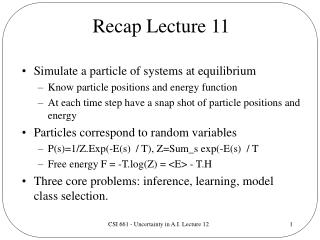# Recap Lecture 11 - PowerPoint PPT PresentationDownload PresentationRecap Lecture 11

Download Presentation## Recap Lecture 11

- - - - - - - - - - - - - - - - - - - - - - - - - - - E N D - - - - - - - - - - - - - - - - - - - - - - - - - - -
##### Presentation Transcript

1. Recap Lecture 11 • Simulate a particle of systems at equilibrium • Know particle positions and energy function • At each time step have a snap shot of particle positions and energy • Particles correspond to random variables • P(s)=1/Z.Exp(-E(s) / T), Z=Sum_s exp(-E(s) / T • Free energy F = -T.log(Z) = <E> - T.H • Three core problems: inference, learning, model class selection. CSI 661 - Uncertainty in A.I. Lecture 12

2. Base Problems To Solve • Calculating expectation of a function • Inference • Optimal Bayesian Classifier • Calculating maximum function value • Finding mode • Multiple modes • Figure 3.1 Neal CSI 661 - Uncertainty in A.I. Lecture 12

3. Stochastic Algorithmic Solutions • Numerical Methods: Exact solutions • Rejection sampling • Importance sampling • Methods to find the posterior mode • Markov Chain Monte Carlo Sampling CSI 661 - Uncertainty in A.I. Lecture 12

4. Markov Chains • Invariant/stationary distributions • Ergodic chains • Irreducible, aperiodic, one stationary distribution. • Computational perspective:  • how quickly moves between states can be generated, • how quickly equilibrium is reached from any initial state, • the number of moves required to move from state x to a state y which is independent of x. CSI 661 - Uncertainty in A.I. Lecture 12

5. Markov Chains • A Markov chain consists of a series of random variables X(0), X(1), X(2), X(3), … X(t) • P(x(t+1) | x(t), x(t-1),x(t-2) … x(0)) = P(x(t+1) | x(t)). • X(0) is known as the initial distribution and the distribution for X(t+1) • We begin in a randomly drawn state from the initial distribution and move around the state space according to the state transition probabilities • Homogenous versus heterogeneous CSI 661 - Uncertainty in A.I. Lecture 12

6. Markov Chain Properties CSI 661 - Uncertainty in A.I. Lecture 12

7. Markov Chain Properties II • It will converge to the equilibrium distribution as t  regardless of X(0) CSI 661 - Uncertainty in A.I. Lecture 12

8. CSI 661 - Uncertainty in A.I. Lecture 12

9. Metropolis Algorithm CSI 661 - Uncertainty in A.I. Lecture 12

10. Hastings Generalization CSI 661 - Uncertainty in A.I. Lecture 12

11. BBN Learning Example CSI 661 - Uncertainty in A.I. Lecture 12

12. Statistical Physics - Background • Micro-state versus Macro-state • Micro-state unknown, partial knowledge • P(s) = 1/Z . Exp(-E(s) / T), Z = Sum_s exp(-E(s) / T • Known as the Gibbs, Boltzmann, canonical, equilibrium distribution • Equivalent to what? • Intensive versus Extensive quantities (grow linearly with system size) • Extensive quantities per unit/particle reach constant limits • Interested in systems at thermodynamic equilibrium • Macroscopic properties can be expressed as expectations CSI 661 - Uncertainty in A.I. Lecture 12

13. Ising Spin Glass Model Phase transitions CSI 661 - Uncertainty in A.I. Lecture 12

14. Free Energy of a SystemRelationship to A.I. • Free energy F = -T.log(Z) = <E> - T.H • Z is the partition function, T temperature • H the system entropy • F and H are extensive values • (cf. Slide 1) What are the analagous particles for: • Parameter estimation (learning) • Inference • Model class selection CSI 661 - Uncertainty in A.I. Lecture 12

15. Next Lecture • Read, Neal, Chapter 2 CSI 661 - Uncertainty in A.I. Lecture 12